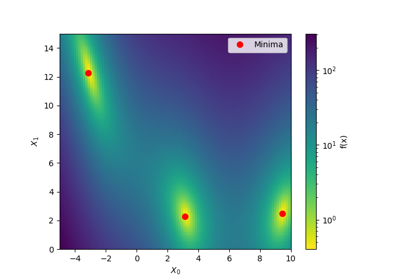# skopt.plots.plot_evaluations¶

skopt.plots.plot_evaluations(result, bins=20, dimensions=None, plot_dims=None)[source][source]

Visualize the order in which points were sampled during optimization.

This creates a 2-d matrix plot where the diagonal plots are histograms that show the distribution of samples for each search-space dimension.

The plots below the diagonal are scatter-plots of the samples for all combinations of search-space dimensions.

The order in which samples were evaluated is encoded in each point’s color.

A red star shows the best found parameters.

Parameters
resultOptimizeResult

The optimization results from calling e.g. gp_minimize().

binsint, bins=20

Number of bins to use for histograms on the diagonal.

dimensionslist of str, default=None

Labels of the dimension variables. None defaults to space.dimensions[i].name, or if also None to ['X_0', 'X_1', ..].

plot_dimslist of str and int, default=None

List of dimension names or dimension indices from the search-space dimensions to be included in the plot. If None then use all dimensions except constant ones from the search-space.

Returns
axMatplotlib.Axes

A 2-d matrix of Axes-objects with the sub-plots.

## Examples using skopt.plots.plot_evaluations¶